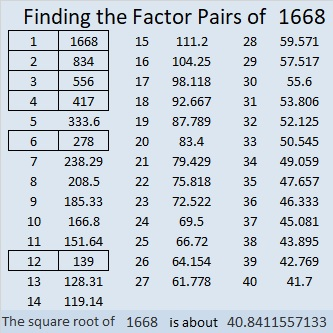# 1668 and Level 5

### Today’s Puzzle:

Write the numbers 1 to 12 in both the first column and the top row so that those numbers and the given clues create a multiplication table. This is a level 5 puzzle, so some of the clues may be tricky. Remember to use logic and consider all possibilities for factors to avoid being tricked.### Factors of 1668:

• 1668 is a composite number.
• Prime factorization: 1668 = 2 × 2 × 3 × 139, which can be written 1668 = 2² × 3 × 139.
• 1668 has at least one exponent greater than 1 in its prime factorization so √1668 can be simplified. Taking the factor pair from the factor pair table below with the largest square number factor, we get √1668 = (√4)(√417) = 2√417.
• The exponents in the prime factorization are 2, 1, and 1. Adding one to each exponent and multiplying we get (2 + 1)(1 + 1)(1 + 1) = 3 × 2 × 2 = 12. Therefore 1668 has exactly 12 factors.
• The factors of 1668 are outlined with their factor pair partners in the graphic below.### More About the Number 1668:

1668 is the difference of two squares in two different ways:
418² – 416² = 1668, and
142² – 136² = 1668.